/* styles */

# Upcoming Important Dates

Tuesday, March 3 - Special LSC Meeting
Friday, March 5 - First Friday
Monday, March 8 - Deadline for Student Artwork for FON Spring Benefit
Tuesday, March 9 - Financial Aid Presentation for Juniors and Sophomores
Miércoles 31 de Marzo - Hora de Conversación en Español

 table div table+table+table+table+table div table{width:100%;padding:0}table div table+table+table+table+table div table img{width:96.23%;padding:0;float:none}table div table+table+table+table+table div table td{width:100%;padding:0 1.88% 18px}/* styles */## News from the Counseling Office

▪ Financial Aid Presentation Planned for Juniors and Sophomores on Tuesday, March 9
▪ College Counseling Articles
▪ Virtual College Fairs
▪ Summer Enrichment/Pre-College Programs
▪ Class of 2022 - Important Dates
▪ Update: Postsecondary Representative Visits
▪ Counseling Department: ILP Naviance Curriculum
 ▪ Financial Aid Presentation Planned for Juniors and Sophomores on Tuesday, March 9
 ▪ College Counseling Articles
 ▪ Virtual College Fairs
 ▪ Summer Enrichment/Pre-College Programs
 ▪ Class of 2022 - Important Dates
 ▪ Update: Postsecondary Representative Visits
 ▪ Counseling Department: ILP Naviance Curriculum

## Sports, Yearbook & General School News

▪ Winter Sports are Back! Events This Week
▪ Sports Update
▪ Available Now: Custom NCP Brick With Your Personalized Message for Your Student
▪ Photo Submissions Requested for NCP Stampede!
▪ Yearbook Ads for Seniors Available
▪ Update on School Portraits for 9th, 10th, and 11th Graders
▪ Order Your 2020-2021 NCP Yearbook - Today is the LAST Day to Get It at a Reduced Rate!
▪ Ways for Parents to Stay in Touch/Volunteer/Support NCP/Social Media Links
 ▪ Winter Sports are Back! Events This Week
 ▪ Sports Update
 ▪ Available Now: Custom NCP Brick With Your Personalized Message for Your Student
 ▪ Photo Submissions Requested for NCP Stampede!
 ▪ Yearbook Ads for Seniors Available
 ▪ Update on School Portraits for 9th, 10th, and 11th Graders
 ▪ Order Your 2020-2021 NCP Yearbook - Today is the LAST Day to Get It at a Reduced Rate!
 ▪ Ways for Parents to Stay in Touch/Volunteer/Support NCP/Social Media Links
 table div table+table+table+table+table+table+table div table{width:100%;padding:0}table div table+table+table+table+table+table+table div table img{width:96.23%;padding:0;float:none}table div table+table+table+table+table+table+table div table td{width:100%;padding:0 1.88% 18px}/* styles */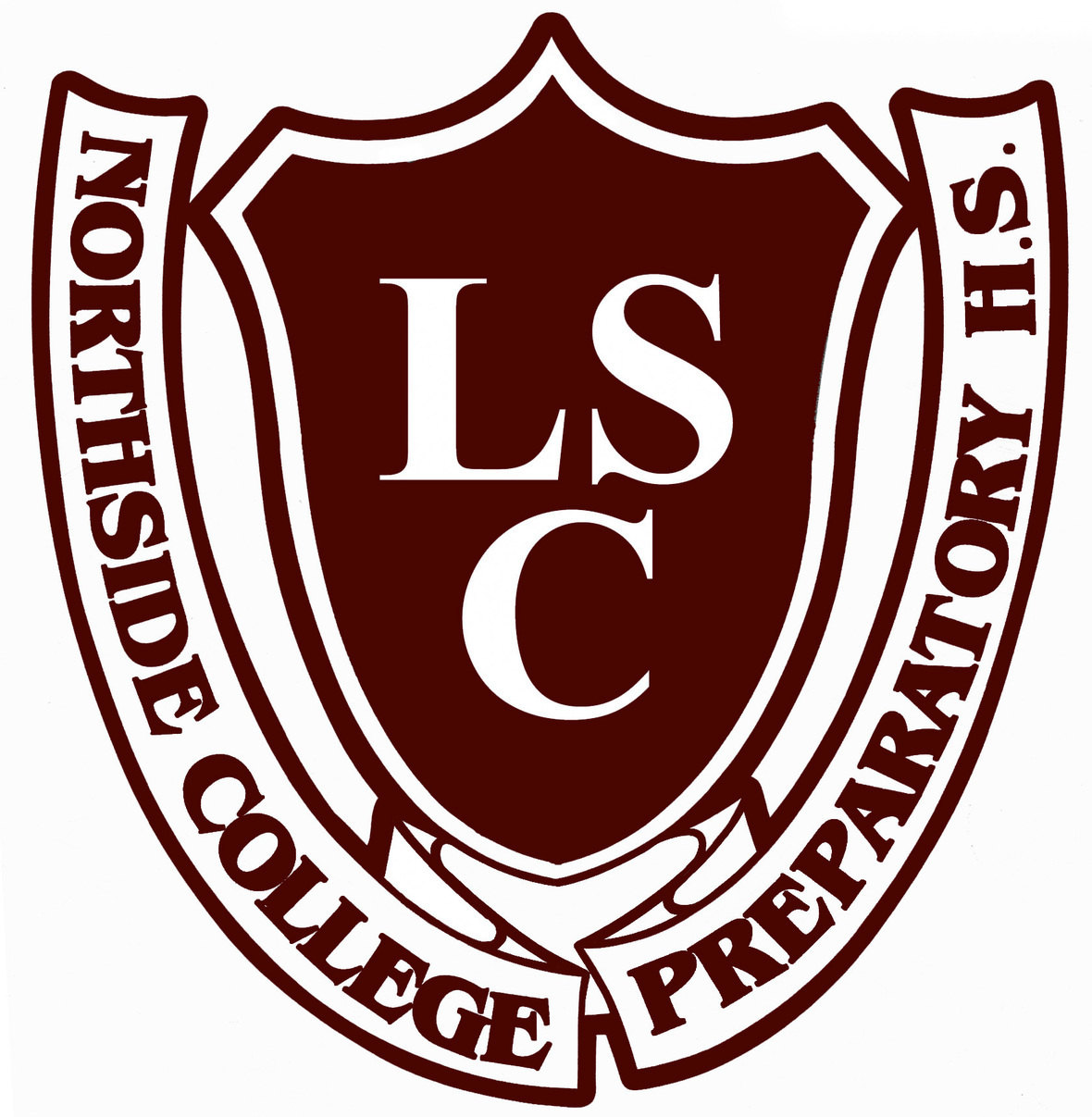# LSC Special Meeting Notice and Agenda

Tuesday, March 3
5:30 pm Zoom Meeting

Both the Zoom link and the agenda can be found on the Northside website here.

https://us02web.zoom.us/j/81084372887?pwd=OWh0bTZHdDlFSTRsYTFJK3l0Nnhudz09

Meeting ID: 810 8437 2887
Passcode: 599109
One tap mobile

+13126266799,,81084372887# US (Chicago)

 table div table+table+table+table+table+table+table+table+table div table{width:100%;padding:0}table div table+table+table+table+table+table+table+table+table div table img{width:96.23%;padding:0;float:none}table div table+table+table+table+table+table+table+table+table div table td{width:100%;padding:0 1.88% 18px}/* styles */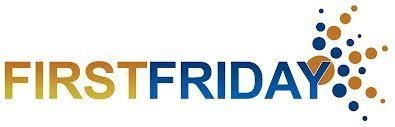# First Friday Virtual Gathering

Friday, March 5
9:00 am Zoom Meeting

Each month, NCP parents and guardians are invited to get together for a monthly coffee gathering. This month, join fellow NCP parents online. Stay for as long as your schedule allows.

Meeting ID: 848 7451 7349
Passcode: 336446
One tap mobile
+13126266799,,84874517349#,,,,336446# US (Chicago)
+19294362866,,84874517349#,,,,
336446# US (New York)

+1 312 626 6799 US (Chicago)

 table div table+table+table+table+table+table+table+table+table+table+table div table{width:100%;padding:0}table div table+table+table+table+table+table+table+table+table+table+table div table img{width:96.23%;padding:0;float:none}table div table+table+table+table+table+table+table+table+table+table+table div table td{width:100%;padding:0 1.88% 18px}/* styles */# FON Spring Benefit Call for Student Artwork: Deadline for Submissions Extended to March 8

Friends of Northside is looking for NCP students to help them visually illustrate their Spring Virtual Fundraising Event theme: "Together but Apart." The past year has been one like no other, and they would like to showcase the resilience, strength, and resourcefulness that our students, faculty, and parents have demonstrated and continue to make Northside a place that exemplifies what is possible in public education.

Students have been asked via NCP email to submit their creative interpretation of the event theme, “Together but Apart,” as a two-dimensional drawing, painting, computer illustration, collage, or other medium that can be scanned flat and sent as a high-resolution color 300dpi jpg, pdf, or eps file. Selected artwork will be featured in marketing materials and communications before and during the event.

The email contains a Google Form to submit their artwork. The deadline for submissions is now Monday, March 8.

 table div table+table+table+table+table+table+table+table+table+table+table+table+table+table div table{width:100%;padding:0}table div table+table+table+table+table+table+table+table+table+table+table+table+table+table div table img{width:96.23%;padding:0;float:none}table div table+table+table+table+table+table+table+table+table+table+table+table+table+table div table td{width:100%;padding:0 1.88% 18px}/* styles */# Hora de Conversación en Español

Miércoles 31 de Marzo
7:00 pm

Se llevará a cabo el miércoles 31 de marzo a las 7:00 pm.

 table div table+table+table+table+table+table+table+table+table+table+table+table+table+table+table+table div table{width:100%;padding:0}table div table+table+table+table+table+table+table+table+table+table+table+table+table+table+table+table div table img{width:96.23%;padding:0;float:none}table div table+table+table+table+table+table+table+table+table+table+table+table+table+table+table+table div table td{width:100%;padding:0 1.88% 18px}/* styles */# Financial Aid Presentation Planned for Juniors and Sophomores on Tuesday, March 9

Hello, Junior and Sophomore Parents,

On Tuesday, March 9, from 6:00 pm - 8:00 pm, the Northside College Prep Counseling Department will host a virtual financial aid presentation for students and parents and/or guardians.

This presentation is being offered to help your family prepare to navigate the financial aid process. During the presentation, the presenters will outline the financial aid process for the Free Application for Federal Student Aid (FAFSA), R.I.S.E. Application for undocumented and transgendered students, and need-based financial aid.

To ensure we are meeting the needs of the Northside Prep community, we are offering presentations in English and Spanish. Click here to register for the virtual financial aid presentation. Once we receive your registration, you will receive a Google Meeting invitation by Friday, March 6.

If you have any questions or concerns, feel free to contact Ms. Moore at mcmoore6@cps.edu.

 table div table+table+table+table+table+table+table+table+table+table+table+table+table+table+table+table+table+table+table div table{width:100%;padding:0}table div table+table+table+table+table+table+table+table+table+table+table+table+table+table+table+table+table+table+table div table img{width:96.23%;padding:0;float:none}table div table+table+table+table+table+table+table+table+table+table+table+table+table+table+table+table+table+table+table div table td{width:100%;padding:0 1.88% 18px}/* styles */# College Counseling Articles

Hello, Class of 2022 Parents,

As your family prepares for your upcoming college conferences, here are a few articles that will prepare you to support your rising senior to navigate the college applications and admissions process. Also, please review the College Planning guide that was emailed to your family and that is listed in the FON newsletter as well.

If you have any questions or concerns, do not hesitate to contact Ms. Moore at mcmoore6@cps.edu or your student’s respective school counselor. We look forward to supporting your family with navigating the next steps in the college admissions process!

▪ Common App Revises Application Questions on Sex and Gender (click here)

 table div table+table+table+table+table+table+table+table+table+table+table+table+table+table+table+table+table+table+table+table+table+table div table{width:100%;padding:0}table div table+table+table+table+table+table+table+table+table+table+table+table+table+table+table+table+table+table+table+table+table+table div table img{width:96.23%;padding:0;float:none}table div table+table+table+table+table+table+table+table+table+table+table+table+table+table+table+table+table+table+table+table+table+table div table td{width:100%;padding:0 1.88% 18px}/* styles */# Virtual College Fairs

Hello Northside Parents,

Due to the global pandemic and the CPS re-opening guidance, Northside College Prep will not host our annual Joint College Fair with Von Steuben High School and Disney II Magnet High School this year.

However, the counseling department compiled a list of virtual postsecondary events for your family to explore.

Northside Prep Virtual Information Sessions
Naviance Virtual College Events
▪ Virtual information sessions and tours (visit college websites for more details)
▪ In-Person College Visits
▪ Virtual College Fairs
IACAC
NACAC
College Board: Big Future Days
CPS College Virtual Field Trips
Gap Year Fairs

 table div table+table+table+table+table+table+table+table+table+table+table+table+table+table+table+table+table+table+table+table+table+table+table+table+table div table{width:100%;padding:0}table div table+table+table+table+table+table+table+table+table+table+table+table+table+table+table+table+table+table+table+table+table+table+table+table+table div table img{width:96.23%;padding:0;float:none}table div table+table+table+table+table+table+table+table+table+table+table+table+table+table+table+table+table+table+table+table+table+table+table+table+table div table td{width:100%;padding:0 1.88% 18px}/* styles */# Summer Enrichment/Pre-College Programs

NCP Parents,

Are you and your student interested in learning about enrichment opportunities or pre-college programs to engage in during the spring or summer? Would you like to build your resume and enhance your academic, personal, or social skills? If the answer is yes, consider reviewing the following resources to assist your family with identifying the best-fit enrichment opportunities for your student:

 table div table+table+table+table+table+table+table+table+table+table+table+table+table+table+table+table+table+table+table+table+table+table+table+table+table+table+table+table div table{width:100%;padding:0}table div table+table+table+table+table+table+table+table+table+table+table+table+table+table+table+table+table+table+table+table+table+table+table+table+table+table+table+table div table img{width:96.23%;padding:0;float:none}table div table+table+table+table+table+table+table+table+table+table+table+table+table+table+table+table+table+table+table+table+table+table+table+table+table+table+table+table div table td{width:100%;padding:0 1.88% 18px}/* styles */## Class of 2022 - Important Dates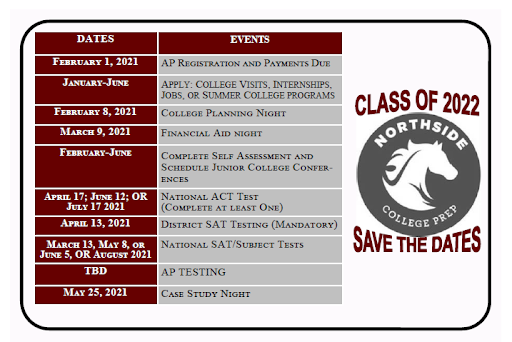table div table+table+table+table+table+table+table+table+table+table+table+table+table+table+table+table+table+table+table+table+table+table+table+table+table+table+table+table+table+table+table div table{width:100%;padding:0}table div table+table+table+table+table+table+table+table+table+table+table+table+table+table+table+table+table+table+table+table+table+table+table+table+table+table+table+table+table+table+table div table img{width:96.23%;padding:0;float:none}table div table+table+table+table+table+table+table+table+table+table+table+table+table+table+table+table+table+table+table+table+table+table+table+table+table+table+table+table+table+table+table div table td{width:100%;padding:0 1.88% 18px}/* styles */# Counseling Department: ILP Naviance Curriculum

The CPS Office of School Counseling and Postsecondary Advising has created quarterly newsletters to continue to engage and support students and parents with their postsecondary planning, including: self-discovery, academic planning, and career and college exploration.

To view the newsletters for each grade level click on the links below. To access the counseling department college planning curriculum, students and parents can log into their Naviance account here to review and complete each task.

 table div table+table+table+table+table+table+table+table+table+table+table+table+table+table+table+table+table+table+table+table+table+table+table+table+table+table+table+table+table+table+table+table+table div table{width:100%;padding:0}table div table+table+table+table+table+table+table+table+table+table+table+table+table+table+table+table+table+table+table+table+table+table+table+table+table+table+table+table+table+table+table+table+table div table img{width:96.23%;padding:0;float:none}table div table+table+table+table+table+table+table+table+table+table+table+table+table+table+table+table+table+table+table+table+table+table+table+table+table+table+table+table+table+table+table+table+table div table td{width:100%;padding:0 1.88% 18px}/* styles */# CPS Scholarship Notifications and College Events Around the City

For more details about upcoming events and to access the most recent updates, click HERE to view the College Calendar on the Northside Prep Counseling website. Also, for additional scholarship opportunities, click HERE to visit the Northside College Prep Counseling page.

 table div table+table+table+table+table+table+table+table+table+table+table+table+table+table+table+table+table+table+table+table+table+table+table+table+table+table+table+table+table+table+table+table+table+table+table div table{width:100%;padding:0}table div table+table+table+table+table+table+table+table+table+table+table+table+table+table+table+table+table+table+table+table+table+table+table+table+table+table+table+table+table+table+table+table+table+table+table div table img{width:96.23%;padding:0;float:none}table div table+table+table+table+table+table+table+table+table+table+table+table+table+table+table+table+table+table+table+table+table+table+table+table+table+table+table+table+table+table+table+table+table+table+table div table td{width:100%;padding:0 1.88% 18px}/* styles */# Winter Sports are Back!/Events This Week

At long last, the winter sports season began last week for our Mustang student-athletes, with the JV and Varsity girls basketball teams making their debut against Taft at NCP last Thursday, followed by the boys’ teams against Lakeview and Von Steuben on Friday and Saturday.

## Upcoming events this week include:

Boys Swimming
▪ Monday, March 3
NCP vs. Whitney Young (away) at 5:00 pm

▪ Monday, March 1 vs. Roosevelt (away) at 5:00 pm
▪ Wednesday, March 3 vs. Roosevelt (away) at 5:00 pm
▪ Friday, March 5 vs. Steinmetz @ NCP at 5:00 pm

▪ Wednesday, March 3 vs. Sullivan (away) (Soph. at 5:00 pm; V at 7:00 pm)
▪ Friday, March 5 vs. Mather (away) (Soph. at 5:00 pm; V at 7:00 pm)
▪ Saturday, March 6 vs. Taft @ NCP (Soph. at 11:30 am; V at 2:00 pm)
▪ Saturday, March 6 vs. Clemente @ NCP (Freshman at 9:00 am)

▪ Tuesday, March 2 vs. Lane Tech (away) (JV at 5:00 pm; V at 7:00 pm)
▪ Thursday, March 4 vs. Jones @ NCP (JV at 5:00 pm; V at 7:00 pm)

Boys and Girls Bowling
▪ Tuesday, March 2 vs. Payton @ Fireside Bowl (5:00 pm)

## Videographers Needed

Unfortunately, under CPS and Illinois safety protocols for indoor sports, there are no spectators allowed in the gyms this season. But in true Mustang spirit, the home opener games for both Girls Basketball teams were live-streamed for parents to view online. A huge thanks to parents Neal Wilson and Rick Petrillo for making that happen.

AD Henderson has indicated that he is open to having parent volunteers record or live-stream sporting events in this non-traditional season (one per game for safety reasons). If any parents would like to volunteer to film any home games, matches, or meets on their iPad or video camera, please let Mr. Henderson know in advance by contacting him at hhenderson@cps.edu, or get in touch with Mike Hughes at mhughes@nge.com to coordinate. It’s a great way to keep other parents, students, and fans connected to the school community, from the comfort of home . . . where the popcorn is free!

 table div table+table+table+table+table+table+table+table+table+table+table+table+table+table+table+table+table+table+table+table+table+table+table+table+table+table+table+table+table+table+table+table+table+table+table+table+table div table{width:100%;padding:0}table div table+table+table+table+table+table+table+table+table+table+table+table+table+table+table+table+table+table+table+table+table+table+table+table+table+table+table+table+table+table+table+table+table+table+table+table+table div table img{width:96.23%;padding:0;float:none}table div table+table+table+table+table+table+table+table+table+table+table+table+table+table+table+table+table+table+table+table+table+table+table+table+table+table+table+table+table+table+table+table+table+table+table+table+table div table td{width:100%;padding:0 1.88% 18px}/* styles */# Sports Update

Keep an eye on the NCP Athletics webpage for updates on spring sports. Boys soccer and women’s volleyball, which were postponed from the fall, are starting practices in the next two weeks, and game schedules will be posted online when they are available.

Traditional spring sports, including lacrosse, softball, baseball, water polo, girls soccer, and boys tennis will begin in early April. Stay tuned for more information.

## Sports Registration

Visit the NCP athletics website HERE and click on the Activity Registration tab. In addition to registering, students must have a current physical on file before they try out or practice. Contact athletic director Henry Henderson at hhenderson@cps.edu for more information.

The athletic department will publish tryout and practice information as it becomes available. Be sure to look for a message via SchoolCNXT and check the NCP athletic website HERE for any updates.

 table div table+table+table+table+table+table+table+table+table+table+table+table+table+table+table+table+table+table+table+table+table+table+table+table+table+table+table+table+table+table+table+table+table+table+table+table+table+table+table div table{width:100%;padding:0}table div table+table+table+table+table+table+table+table+table+table+table+table+table+table+table+table+table+table+table+table+table+table+table+table+table+table+table+table+table+table+table+table+table+table+table+table+table+table+table div table img{width:96.23%;padding:0;float:none}table div table+table+table+table+table+table+table+table+table+table+table+table+table+table+table+table+table+table+table+table+table+table+table+table+table+table+table+table+table+table+table+table+table+table+table+table+table+table+table div table td{width:100%;padding:0 1.88% 18px}/* styles */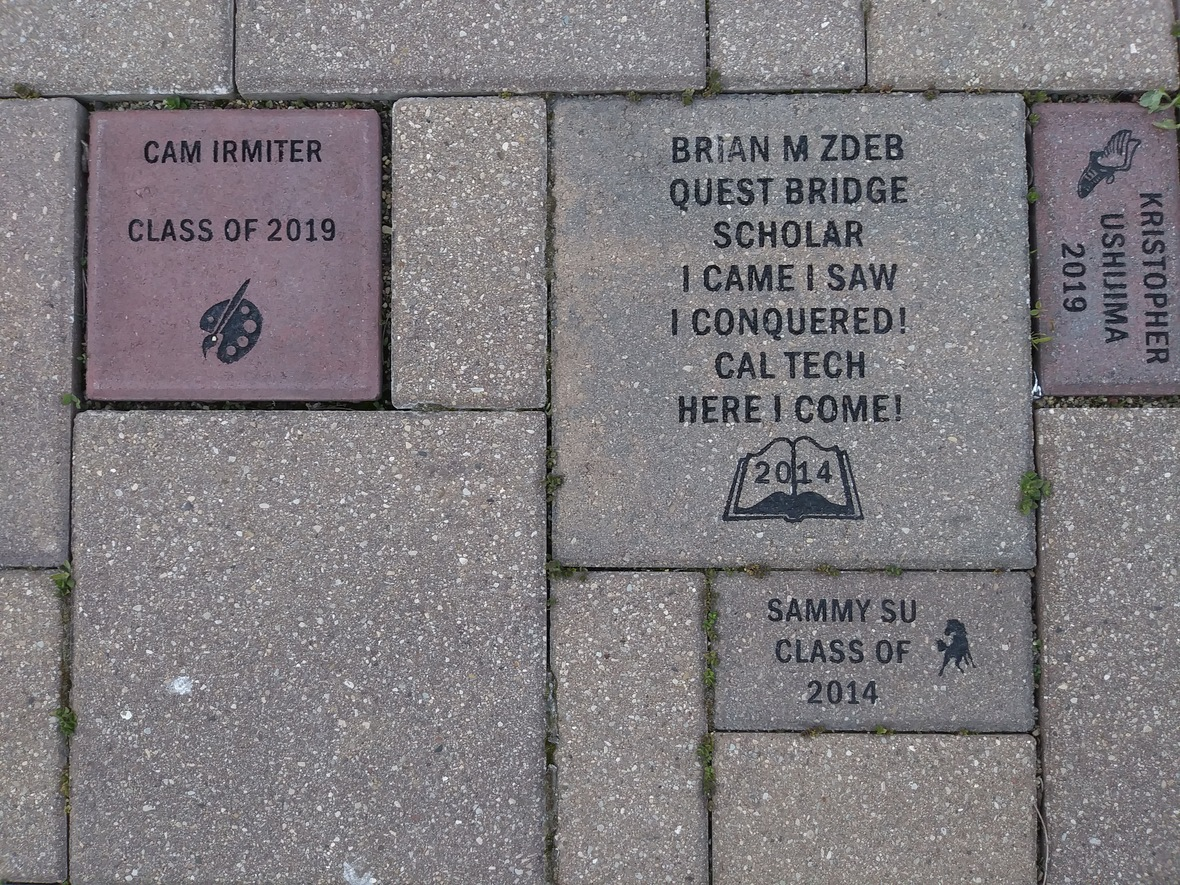Consider buying a custom NCP Brick for your child this year. Your brick, engraved with a personal message, will become a permanent part of the Northside College Prep Celestial Garden Walkway.

Your brick may be purchased in three sizes:

▪ 4" x 8" = \$50
▪ 8" x 8" = \$100
▪ 12" x 12" = \$150

Friends of Northside will provide a gift certificate acknowledging the purchase. Janet Jasmer will assist with the process and can answer any questions at jjasmer@atproperties.com.

 table div table+table+table+table+table+table+table+table+table+table+table+table+table+table+table+table+table+table+table+table+table+table+table+table+table+table+table+table+table+table+table+table+table+table+table+table+table+table+table+table+table+table div table{width:100%;padding:0}table div table+table+table+table+table+table+table+table+table+table+table+table+table+table+table+table+table+table+table+table+table+table+table+table+table+table+table+table+table+table+table+table+table+table+table+table+table+table+table+table+table+table div table img{width:96.23%;padding:0;float:none}table div table+table+table+table+table+table+table+table+table+table+table+table+table+table+table+table+table+table+table+table+table+table+table+table+table+table+table+table+table+table+table+table+table+table+table+table+table+table+table+table+table+table div table td{width:100%;padding:0 1.88% 18px}/* styles */# Any Questions About NCP Communications Sent Via CNXT?

If you are having problems with SchoolCNXT, please contact the main office from 7:30 am - 3:15 pm at 773-534-3954, or you can email the NCP school clerk, Mary Grasz, at mtgrasz@cpu.edu.

NCP uses SchoolCNXT to communicate important information to parents and the school community. All families should have received a text message and/or email communication about signing up. If you did not receive that communication or need access to your student's account, you can gain access by using this tool on Northside's website.

 table div table+table+table+table+table+table+table+table+table+table+table+table+table+table+table+table+table+table+table+table+table+table+table+table+table+table+table+table+table+table+table+table+table+table+table+table+table+table+table+table+table+table+table+table+table div table{width:100%;padding:0}table div table+table+table+table+table+table+table+table+table+table+table+table+table+table+table+table+table+table+table+table+table+table+table+table+table+table+table+table+table+table+table+table+table+table+table+table+table+table+table+table+table+table+table+table+table div table img{width:96.23%;padding:0;float:none}table div table+table+table+table+table+table+table+table+table+table+table+table+table+table+table+table+table+table+table+table+table+table+table+table+table+table+table+table+table+table+table+table+table+table+table+table+table+table+table+table+table+table+table+table+table div table td{width:100%;padding:0 1.88% 18px}/* styles */# Photo Submissions Requested for NCP Stampede!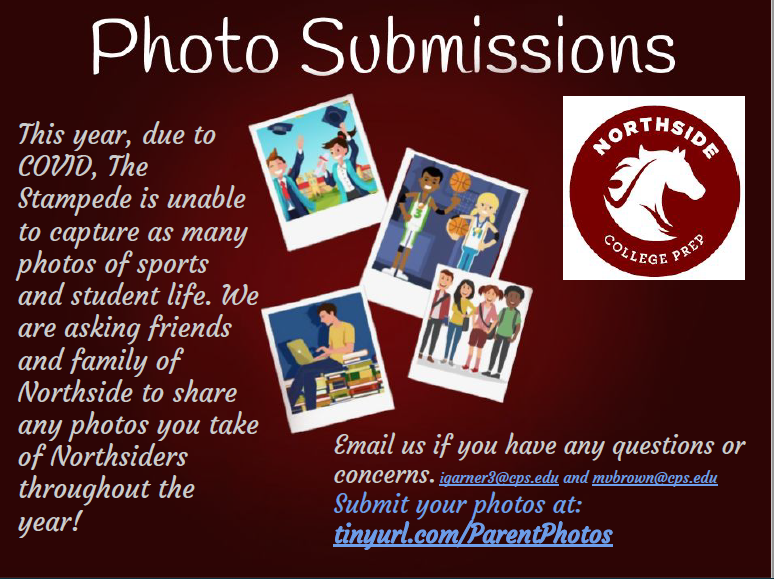# 2020-2021 Yearbook Ads for Seniors Now Available

Seniors now have the opportunity to place ads in the 2020-2021 yearbook by visiting the yearbook website. After clicking on "continue", click on "start your ad" to begin.

 table div table+table+table+table+table+table+table+table+table+table+table+table+table+table+table+table+table+table+table+table+table+table+table+table+table+table+table+table+table+table+table+table+table+table+table+table+table+table+table+table+table+table+table+table+table+table+table+table+table div table td,table.module-48{width:100%;padding:0}table div table+table+table+table+table+table+table+table+table+table+table+table+table+table+table+table+table+table+table+table+table+table+table+table+table+table+table+table+table+table+table+table+table+table+table+table+table+table+table+table+table+table+table+table+table+table+table+table+table div table{width:100%;float:none;margin-left:auto;margin-right:auto;padding:0}table div table+table+table+table+table+table+table+table+table+table+table+table+table+table+table+table+table+table+table+table+table+table+table+table+table+table+table+table+table+table+table+table+table+table+table+table+table+table+table+table+table+table+table+table+table+table+table+table+table div table a{border:0 none;text-decoration:none}table div table+table+table+table+table+table+table+table+table+table+table+table+table+table+table+table+table+table+table+table+table+table+table+table+table+table+table+table+table+table+table+table+table+table+table+table+table+table+table+table+table+table+table+table+table+table+table+table+table div table img{width:100%!important;border:0 none;text-decoration:none}/* styles */

# Update on School Portraits for 9th, 10th, and 11th Graders

This letter was sent in CNXT explaining the process for submitting your student's school portrait to be included in the yearbook. The NCP code is 21740 if needed. If you have any questions, contact Ms. McCreedy at mkmccreedy@cps.edu.

# Today is the Last Day to Order a Yearbook for \$45 - Order a Yearbook Today!

Interested in purchasing a copy of the 2020-2021 Stampede, NCP's yearbook? Click HERE to start your order! And if you didn't get a chance to purchase a copy of the 2019-2020 yearbook, you now have a change to buy one of those, too! Just email Ms. McCreedy at mkmccreedy@cps.edu after you've completed your 2020-2021 order.

Copies are \$45 until March 1 and \$55 afterwards.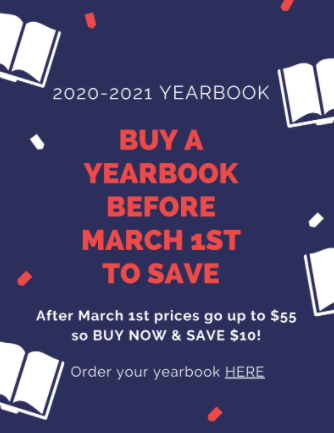table div table+table+table+table+table+table+table+table+table+table+table+table+table+table+table+table+table+table+table+table+table+table+table+table+table+table+table+table+table+table+table+table+table+table+table+table+table+table+table+table+table+table+table+table+table+table+table+table+table+table+table+table+table div table{width:100%;padding:0}table div table+table+table+table+table+table+table+table+table+table+table+table+table+table+table+table+table+table+table+table+table+table+table+table+table+table+table+table+table+table+table+table+table+table+table+table+table+table+table+table+table+table+table+table+table+table+table+table+table+table+table+table+table div table img{width:96.23%;padding:0;float:none}table div table+table+table+table+table+table+table+table+table+table+table+table+table+table+table+table+table+table+table+table+table+table+table+table+table+table+table+table+table+table+table+table+table+table+table+table+table+table+table+table+table+table+table+table+table+table+table+table+table+table+table+table+table div table td{width:100%;padding:0 1.88% 18px}/* styles */# Do you have news or team results to share with the Northside parent community?

 table div table+table+table+table+table+table+table+table+table+table+table+table+table+table+table+table+table+table+table+table+table+table+table+table+table+table+table+table+table+table+table+table+table+table+table+table+table+table+table+table+table+table+table+table+table+table+table+table+table+table+table+table+table+table+table+table div table{width:100%;padding:0}table div table+table+table+table+table+table+table+table+table+table+table+table+table+table+table+table+table+table+table+table+table+table+table+table+table+table+table+table+table+table+table+table+table+table+table+table+table+table+table+table+table+table+table+table+table+table+table+table+table+table+table+table+table+table+table+table div table img{width:96.23%;padding:0;float:none}table div table+table+table+table+table+table+table+table+table+table+table+table+table+table+table+table+table+table+table+table+table+table+table+table+table+table+table+table+table+table+table+table+table+table+table+table+table+table+table+table+table+table+table+table+table+table+table+table+table+table+table+table+table+table+table+table div table td{width:100%;padding:0 1.88% 18px}/* styles */# Support Northside - Shop AmazonSmile

AmazonSmile is a simple and automatic way to support Northside every time you shop.

Please note that our AmazonSmile name is Friends of "North" "Side" (North Side - two words at AmazonSmile).

 table div table+table+table+table+table+table+table+table+table+table+table+table+table+table+table+table+table+table+table+table+table+table+table+table+table+table+table+table+table+table+table+table+table+table+table+table+table+table+table+table+table+table+table+table+table+table+table+table+table+table+table+table+table+table+table+table+table+table div table{width:100%;padding:0}table div table+table+table+table+table+table+table+table+table+table+table+table+table+table+table+table+table+table+table+table+table+table+table+table+table+table+table+table+table+table+table+table+table+table+table+table+table+table+table+table+table+table+table+table+table+table+table+table+table+table+table+table+table+table+table+table+table+table div table img{width:96.23%;padding:0;float:none}table div table+table+table+table+table+table+table+table+table+table+table+table+table+table+table+table+table+table+table+table+table+table+table+table+table+table+table+table+table+table+table+table+table+table+table+table+table+table+table+table+table+table+table+table+table+table+table+table+table+table+table+table+table+table+table+table+table+table div table td{width:100%;padding:0 1.88% 18px}/* styles */# Follow Us on Social Media

 table div table+table+table+table+table+table+table+table+table+table+table+table+table+table+table+table+table+table+table+table+table+table+table+table+table+table+table+table+table+table+table+table+table+table+table+table+table+table+table+table+table+table+table+table+table+table+table+table+table+table+table+table+table+table+table+table+table+table+table+table div table{width:100%;padding:0}table div table+table+table+table+table+table+table+table+table+table+table+table+table+table+table+table+table+table+table+table+table+table+table+table+table+table+table+table+table+table+table+table+table+table+table+table+table+table+table+table+table+table+table+table+table+table+table+table+table+table+table+table+table+table+table+table+table+table+table+table div table img{width:96.23%;padding:0;float:none}table div table+table+table+table+table+table+table+table+table+table+table+table+table+table+table+table+table+table+table+table+table+table+table+table+table+table+table+table+table+table+table+table+table+table+table+table+table+table+table+table+table+table+table+table+table+table+table+table+table+table+table+table+table+table+table+table+table+table+table+table div table td{width:100%;padding:0 1.88% 18px}/* styles */table div table+table+table+table+table+table+table+table+table+table+table+table+table+table+table+table+table+table+table+table+table+table+table+table+table+table+table+table+table+table+table+table+table+table+table+table+table+table+table+table+table+table+table+table+table+table+table+table+table+table+table+table+table+table+table+table+table+table+table+table+table+table div table{width:100%;padding:0}table div table+table+table+table+table+table+table+table+table+table+table+table+table+table+table+table+table+table+table+table+table+table+table+table+table+table+table+table+table+table+table+table+table+table+table+table+table+table+table+table+table+table+table+table+table+table+table+table+table+table+table+table+table+table+table+table+table+table+table+table+table+table div table img{width:96.23%;padding:0;float:none}table div table+table+table+table+table+table+table+table+table+table+table+table+table+table+table+table+table+table+table+table+table+table+table+table+table+table+table+table+table+table+table+table+table+table+table+table+table+table+table+table+table+table+table+table+table+table+table+table+table+table+table+table+table+table+table+table+table+table+table+table+table+table div table td{width:100%;padding:0 1.88% 18px}/* styles */table div table+table+table+table+table+table+table+table+table+table+table+table+table+table+table+table+table+table+table+table+table+table+table+table+table+table+table+table+table+table+table+table+table+table+table+table+table+table+table+table+table+table+table+table+table+table+table+table+table+table+table+table+table+table+table+table+table+table+table+table+table+table+table+table div table{width:100%;padding:0}table div table+table+table+table+table+table+table+table+table+table+table+table+table+table+table+table+table+table+table+table+table+table+table+table+table+table+table+table+table+table+table+table+table+table+table+table+table+table+table+table+table+table+table+table+table+table+table+table+table+table+table+table+table+table+table+table+table+table+table+table+table+table+table+table div table img{width:96.23%;padding:0;float:none}table div table+table+table+table+table+table+table+table+table+table+table+table+table+table+table+table+table+table+table+table+table+table+table+table+table+table+table+table+table+table+table+table+table+table+table+table+table+table+table+table+table+table+table+table+table+table+table+table+table+table+table+table+table+table+table+table+table+table+table+table+table+table+table+table div table td{width:100%;padding:0 1.88% 18px}/* styles */